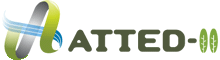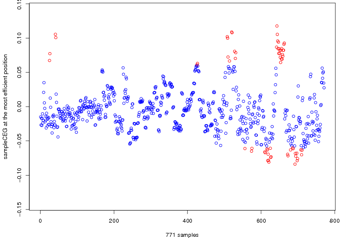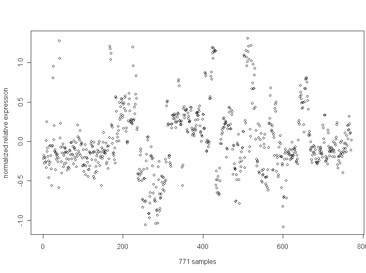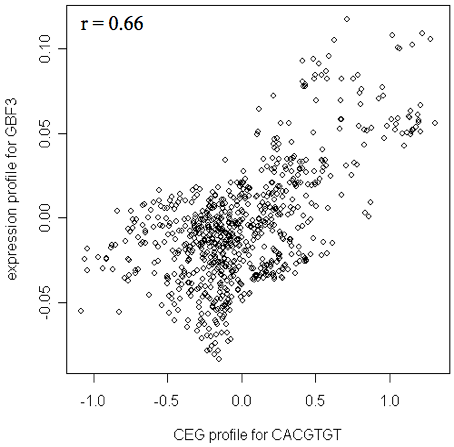#ver.11.1

last update; Jun. 25. 2019

## Prediction of the TF binding a cis element using CEG

### CEG profile of a cis element

The prediction of the transcription factor (TF) binding a cis element is based on the correlation between the CEG profile of a cis element and the gene expression profile of TF.
Figure 1 is the CEG profile of "CACGTGT". The 771 array samples are given on the x-axis. The y-axis is the CEG values at the most efficient position CEG (c="CACGTGT", s, p=(the most effective position)).Figure 1

### Gene expression profile of a TF

Figure 2 is the expression profile of a TF, GBF3. The 771 array samples are given on the x-axis. The y-axis is the normalized relative expression to average level of this gene.Figure 2

### Correlation between a cis element and a TF

Both profiles for cis element and TF include 771 array samples. On the figure below, 771 samples are plotted. The x-axis is the CEG value for CACGTGT, and the y-axis is the relative gene expression of GBF3.When this TF's expression level increases, the CEG value of this cis element candidate also increases. The Pearson's correlation coefficient is 0.66.
In cis element page, TFs with a Pearson's correlation coefficient against the cis element higher than 0.5 or lower than -0.5 are listed.
A positive correlation suggests an activator, while a negative correlation suggests a repressor.

List of predicted TFs whose mRNA profiles are correlated (|r| > 0.5) with CEG profile of CACGTGT.
CorrelTFFunction*((alias))
0.71At4g27410no apical meristem (NAM) family protein (RD26) ((RD26))
0.67At1g01720no apical meristem (NAM) family protein ((ATAF1))
0.66At2g46270G-box binding factor 3 (GBF3) ((GBF3))
0.62At5g12840CCAAT-binding transcription factor (CBF-B/NF-YA) family protein ((HAP2A))
0.60At1g77450no apical meristem (NAM) family protein
.........
[Link to cis element page of CACGTGT]